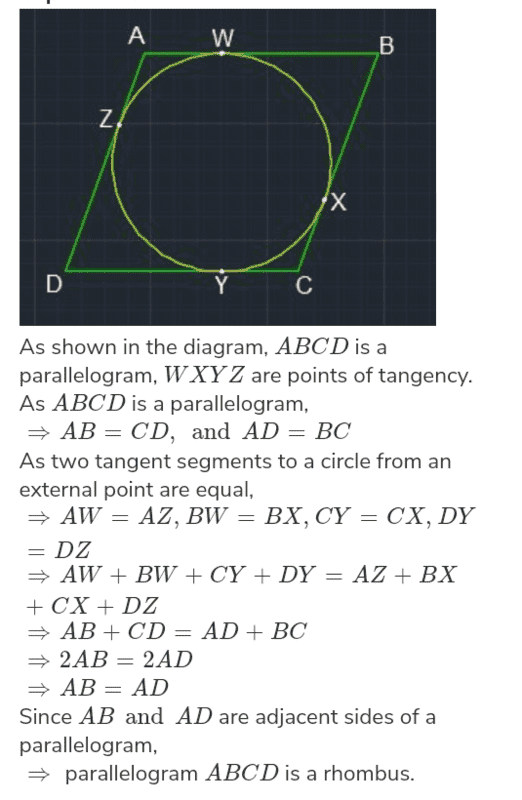# A math problem related to circles

• STEM_nerd
Not me though, I couldn't understand what it means by "change". My first thought was "How does it start and how does it change ", "before or after the inscribe"f

#### STEM_nerd

New user has been reminded to post schoolwork problems in the Homework Help forums
Summary:: If a circle can be inscribed in a parallelogram how will the parallelogram change? Explain.

It is a 10th grade math question in case you want to know.

Summary:: If a circle can be inscribed in a parallelogram how will the parallelogram change? Explain.
The question is unclear. In order for the parallelogram to "change", it would need to have some original shape. No original shape is mentioned in the question.

What does it mean to inscribe a circle in a shape like a parallogram?

Since this is a homework like problem, we can't simply answer it but can only provide hints to help you solve. My question is your first hint.

•STEM_nerd
What does it mean to inscribe a circle in a shape like a parallogram?

Since this is a homework like problem, we can't simply answer it but can only provide hints to help you solve. My question is your first hint.

I got the answer now! It will become a rhombus.

•PeroK
The question is unclear. In order for the parallelogram to "change", it would need to have some original shape. No original shape is mentioned in the question.

The question here refers to the classic parallelogram which has equal opposite angles and equal opposite and parallel sides. It doesn't mean special parallelograms like square, rectangle, rhombus and kite.

•jedishrfu
If a circle can be inscribed in a parallelogram how will the parallelogram change? Explain.
This problem makes no sense, as written. And the solution you gave, that it will become a rhombus, also makes no sense.
I suspect that there is additional information that hasn't been presented here.

•This problem makes no sense, as written. And the solution you gave, that it will become a rhombus, also makes no sense.
I suspect that there is additional information that hasn't been presented here.
There is no additional information given. The only additional thing is the diagram. That's all that's given in the question bank.
My solution that it's a rhombus definitely makes a lot of sense.
Here's the proof that I came up with.Last edited:
•Chestermiller and Delta2
Here's the original wording:
If a circle can be inscribed in a parallelogram how will the parallelogram change?
Your solution looks fine to me, but the wording above suggests that the parallelogram will be undergoing some change, and that's what threw me and several others off.
A better wording for the problem might be:
"If a circle can be inscribed in a parallelogram, what conditions does this fact impose on the parallelogram?"

•Delta2 and DaveC426913
Here's the original wording:

Your solution looks fine to me, but the wording above suggests that the parallelogram will be undergoing some change, and that's what threw me and several others off.
A better wording for the problem might be:
"If a circle can be inscribed in a parallelogram, what conditions does this fact impose on the parallelogram?"
Your confusion is understandable. But that's exactly what was written in the question. It threw me off at first too. And thank you for suggesting a better wording for the question.

Your confusion is understandable. But that's exactly what was written in the question. It threw me off at first too. And thank you for suggesting a better wording for the question.
That's funny. The first thing I thought of is that it has to be a rhombus.

•STEM_nerd
That's funny. The first thing I thought of is that it has to be a rhombus.
Not me though, I couldn't understand what it means by "change". My first thought was "How does it start and how does it change ", "before or after the inscribe"

•STEM_nerd
Maybe it's “how would your word for it change?"